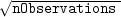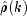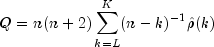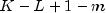JMSLTM Numerical Library 7.2.0
com.imsl.stat

## Class LackOfFit

• ```public class LackOfFit
extends Object```
Performs lack-of-fit test for a univariate time series or transfer function given the appropriate correlation function.

`LackOfFit` may be used to diagnose lack of fit in both ARMA and transfer function models. Typical arguments for these situations are:

 Model `lagMin` `lagMax` `npFree` ARMA (p, q) 1p + q Transfer function 0r + s

`LackOfFit` performs a portmanteau lack of fit test for a time series or transfer function containing `nObservations` observations given the appropriate sample correlation functionfor k = L, L+1,...,K where L = `lagMin` and K = `lagMax`.

The basic form of the test statistic Q iswith L = 1 ifis an autocorrelation function. Given that the model is adequate, Q has a chi-squared distribution withdegrees of freedom where m = `npFree` is the number of parameters estimated in the model. If the mean of the time series is estimated, Woodfield (1990) recommends not including this in the count of the parameters estimated in the model. Thus, for an ARMA(p, q) model set `npFree` = p + q regardless of whether the mean is estimated or not. The original derivation for time series models is due to Box and Pierce (1970) with the above modified version discussed by Ljung and Box (1978). The extension of the test to transfer function models is discussed by Box and Jenkins (1976, pages 394-395).

Example
• ### Method Summary

Methods
Modifier and Type Method and Description
`static double[]` ```compute(int nObservations, double[] correlations, int npFree, int lagMax)```
Performs lack-of-fit test for a univariate time series or transfer function given the appropriate correlation function using a minimum lag of 1.
`static double[]` ```compute(int nObservations, double[] correlations, int npFree, int lagMax, int lagMin)```
Performs lack-of-fit test for a univariate time series or transfer function given the appropriate correlation function.
• ### Methods inherited from class java.lang.Object

`clone, equals, finalize, getClass, hashCode, notify, notifyAll, toString, wait, wait, wait`
• ### Method Detail

• #### compute

```public static double[] compute(int nObservations,
double[] correlations,
int npFree,
int lagMax)```
Performs lack-of-fit test for a univariate time series or transfer function given the appropriate correlation function using a minimum lag of 1.
Parameters:
`nObservations` - an `int` containing the number of observations of the stationary time series.
`correlations` - a `double` array of length ```lagMax+1 ``` containing the correlation function.
`npFree` - an `int` scalar specifying the number of free parameters in the formulation of the time series model. `npfree` must be greater than or equal to zero and less than `lagMax`. Woodfield (1990) recommends `npFree = p + q`.
`lagMax` - an `int` scalar specifying the maximum lag of the correlation function.
Returns:
a `double` array of length 2 with the test statistic, Q, and its p-value, p. Under the null hypothesis, Q has an approximate chi-squared distribution with `lagMax-lagMin+1-npFree` degrees of freedom.
• #### compute

```public static double[] compute(int nObservations,
double[] correlations,
int npFree,
int lagMax,
int lagMin)```
Performs lack-of-fit test for a univariate time series or transfer function given the appropriate correlation function.
Parameters:
`nObservations` - an `int` containing the number of observations of the stationary time series.
`correlations` - a `double` array of length ```lagMax+1 ``` containing the correlation function.
`npFree` - an `int` scalar specifying the number of free parameters in the formulation of the time series model. `npfree` must be greater than or equal to zero and less than `lagMax`. Woodfield (1990) recommends `npFree = p + q`.
`lagMax` - an `int` scalar specifying the maximum lag of the correlation function.
`lagMin` - an `int` scalar specifying the minimum lag of the correlation function. `lagMin` corresponds to the lower bound of summation in the lack of fit test statistic. Default value is 1.
Returns:
a `double` array of length 2 with the test statistic, Q, and its p-value, p. Under the null hypothesis, Q has an approximate chi-squared distribution with `lagMax-lagMin+1-npFree` degrees of freedom.
JMSLTM Numerical Library 7.2.0